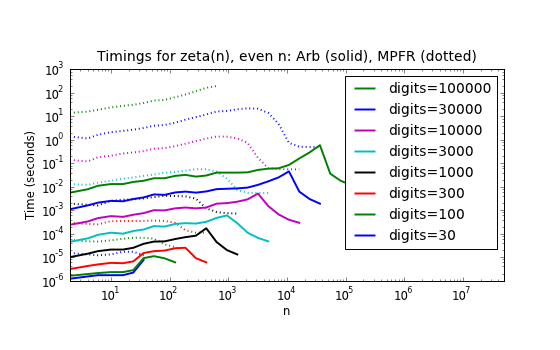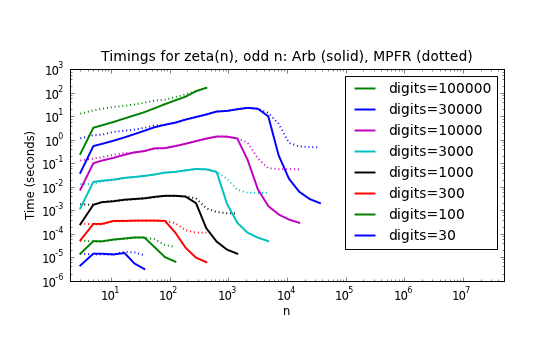# Algorithm selection for zeta(n)

April 19, 2012

In my last post, I mentioned using binary splitting to compute $\zeta(n)$ to extremely high precision for small $n$. I have now added a function to Arb for evaluating $\zeta(n)$ that selects between several different algorithms depending on both $n$ and the precision.

Here are timings compared to MPFR for even $n$:Here the Arb function either uses the formula $\zeta(2n) = (-1)^{n+1} B_{2n} (2\pi)^{2n} / (2(2n)!)$ (computing the Bernoulli number $B_{2n}$ exactly using FLINT), or the Euler product $(\zeta(n))^{-1} = \prod_{p} (1-p^{-n})$ for large $n$. You can see the cutoff quite clearly in the plot. At any size, this strategy is much faster than the generic algorithm used by MPFR.

In fact, since FLINT uses the Euler product to compute Bernoulli numbers, the Euler product effectively always ends up being used. That is, one basically uses the Euler product to compute $\zeta(n)$ to full precision, or to compute $B_n$ exactly, whichever requires less precision.

Timings for odd $n$ look a bit different:There is a visible speedup at very high precision for small $n$ due to the use of binary splitting (timings can be improved further by writing better code for the special cases $n = 5, 7$), and a speedup for asymptotically large $n$ where Arb again uses the Euler product. In between, the code simply falls back to the MPFR function, so the timings are identical.

The pressing question is whether one can do better than MPFR here. Euler-Maclaurin summation might be a bit faster if optimized carefully, but I can’t really think of anything that would make a huge difference.

Another way to compute $\zeta(n)$ to high precision when $n$ is not too large is to use the algorithm of E. Karatsuba. However, my tests so far indicate that it is much slower than other methods in practice.

These timings are all for computing $\zeta(n)$ as an isolated value. Much better performance is possible when computing $\zeta(2), \zeta(3), \ldots, \zeta(n)$ simultaneously. More on that at a later time…